Germán Rodríguez
Survival Analysis Princeton University## Survival with Competing Risks

Singer and Willet (2003) Applied Longitudinal Data Analysis, Oxford, analyze data on the length of service of U.S. Supreme Court Justices, treating death and retirement as competing risks, and age at appointment and calendar year of appointment as predictors.

To conduct a similar analysis I extracted data from Wikipedia and the Supreme Court website, and prepared a comma-separated-value file with the justice number, name, and strings representing the dates of birth, the dates tenure starts and stops, and a status variable that takes the values Incumbent, Died, Resigned or Retired. I ignore the return engagements of John Rutledge and Charles Evans Hughes, who resigned and later were reappointed as Chief Justice.

The file is available on this website as `justices.csv` and can be read as shown in the Stata and R logs. All dates include the day, month and year. The date of birth of James Moore Wayne was available as year only in both sources, so I set the month and day to July 1. All other dates were complete. (An earlier version of this dataset recorded all dates using years only, for simplicity and consistency with the reference. That version is available in Stata 11 format as `justices.dta`. It was last updated in 2016.)

We start by reading the data and converting the dates from strings to the native date format in your statistical package. Note that `stopstr` is empty for incumbents. We set it to February 22, 2018 for these cases, effectively censoring the data as of the date this analysis was run. I calculate `tenure` in years dividing by 365.25. The `event` indicator is coded 0 for current Justices, 1 for those who died while serving, and 2 for those who resigned or retired. I also compute `age` at appointment and `year` of appointment, and save the dataset for later use.

```. import delimited using https://grodri.github.io/datasets/justices.csv, clear
(encoding automatically selected: ISO-8859-1)
(7 vars, 113 obs)

. gen birth = date(birthstr, "MDY")

. gen start = date(startstr, "MDY")

. replace stopstr = "February 22, 2018" if stopstr == ""

. gen stop = date(stopstr, "MDY")

. gen tenure = (stop - start)/365.25

. gen event = (status == "Died") + ///
>     2*(status == "Resigned" | status == "Retired")

. gen age = (start - birth)/365.25

. gen year = year(start) + (doy(start)-1)/doy(mdy(12,31,year(start)))

. drop *str

. save justices2, replace
file justices2.dta saved
```
```> justices <- read.csv("https://grodri.github.io/datasets/justices.csv",
+     stringsAsFactors = FALSE)
> table(justices\$status)

Died Incumbent  Resigned   Retired
50         9        17        37
> library(dplyr)
> library(lubridate)
> map <- data.frame(code=c(0,1,2,2))
> row.names(map) <- c("Incumbent","Died","Resigned","Retired")
> justices <- mutate(justices,
+     birth = mdy(birthstr),
+     start = mdy(startstr),
+     stop  = mdy(ifelse(stopstr=="", "February 22, 2018", stopstr)),
+     tenure = (stop - start)/365.25,
+     event = map[status,],
+     age  = (start - birth)/365.25,
+     year = year(start) +
+         (yday(start)-1)/ifelse(leap_year(year(start)),366,365)) |>
+ select(-ends_with("str"))
> write.csv(justices, file = "justices2.csv")
```

### Overall Survival

The first thing we calculate is the Kaplan-Meier estimator of overall survival

```. stset tenure, fail(event) // event > 0

Survival-time data settings

Failure event: event!=0 & event<.
Observed time interval: (0, tenure]
Exit on or before: failure

──────────────────────────────────────────────────────────────────────────
113  total observations
0  exclusions
──────────────────────────────────────────────────────────────────────────
113  observations remaining, representing
104  failures in single-record/single-failure data
1,887.365  total analysis time at risk and under observation
At risk from t =         0
Earliest observed entry t =         0
Last observed exit t =  36.57221

. sts graph

Failure _d: event
Analysis time _t: tenure

. graph export justices-km.png, width(500) replace
file justices-km.png saved as PNG format

. sts gen km = s // save for later use

. list _t km if abs(km - 0.5) < 0.01

┌───────────────────────┐
│        _t          km │
├───────────────────────┤
15. │ 16.156057   .50834001 │
89. │ 16.490076    .4989263 │
└───────────────────────┘
```
```> library(survival)
> km <- survfit(Surv(tenure, event > 0) ~ 1, data=justices)
> km
Call: survfit(formula = Surv(tenure, event > 0) ~ 1, data = justices)

n events median 0.95LCL 0.95UCL
[1,] 113    104   16.5    14.3    20.3
> source("ggfy.R.txt")
> png("justices-kmr.png", width=500, height=400)
> ggfy(km)
> dev.off()
null device
1
```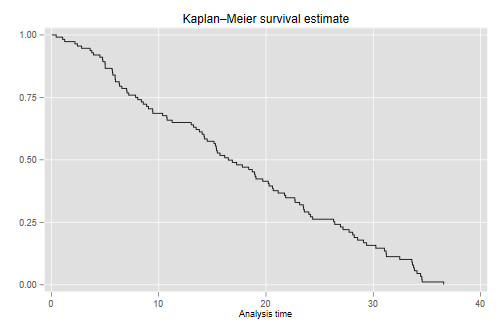The median length of tenure in the court is 16.5 years.

### Incidence Functions

We will use the command `stcompet`, written by V. Coviello and M. Boggess (2004) “Cumulative incidence estimation in the presence of competing risks” Stata Journal, 4(2):103-112, to estimate incidence functions. We specify death as the event of interest and retirement as the competing event. We will use the function `cuminc()` in Bob Grays’s `cmprsk` package to estimate cumulative incidence functions.

```. streset, fail(event == 1)
-> stset tenure, failure(event==1)

Survival-time data settings

Failure event: event==1
Observed time interval: (0, tenure]
Exit on or before: failure

──────────────────────────────────────────────────────────────────────────
113  total observations
0  exclusions
──────────────────────────────────────────────────────────────────────────
113  observations remaining, representing
50  failures in single-record/single-failure data
1,887.365  total analysis time at risk and under observation
At risk from t =         0
Earliest observed entry t =         0
Last observed exit t =  36.57221

. stcompet ci = ci, compet(2) // you just specify the code
```
```> library(cmprsk)
> cif <- cuminc(justices\$tenure, justices\$event)
> cifd <- data.frame(
+     cause = factor(rep(c("death","retirement"),
+             c(length(cif[]\$time), length(cif[]\$time)))),
+     time  = c(cif[]\$time, cif[]\$time),
+     cif   = c(cif[]\$est,  cif[]\$est))
```

The code generates the cumulative incidence functions CIF for each competing cause. To plot them you need to select the appropriate event code. I stacked them into a data frame suitable for use with `ggplot()`. Here’s the incidence of death and retirement

```. twoway line ci _t if event==1, c(J) sort ///
>     title(Cumulative incidence of death) name(d, replace)

. twoway line ci _t if event==2 & _t > 0, c(J) sort ///
>     title(Cumulative incidence of retirement) name(r, replace)

. graph combine d r, xsize(6) ysize(3)

. graph export justices-cif.png, width(720) height(360) replace
file justices-cif.png saved as PNG format
```
```> library(ggplot2)
> g1 <- ggplot(filter(cifd, cause=="death"), aes(time, cif)) +
+     geom_step() + ggtitle("Incidence of Death")
> g2 <- ggplot(filter(cifd, cause=="retirement"), aes(time, cif)) +
+     geom_step() + ggtitle("Incidence of Retirement")
> library(gridExtra)
> g <- arrangeGrob(g1, g2, ncol=2); #plot(g)
> ggsave(plot = g, "justices-cifr.png", width = 10, height = 5, dpi = 72)
```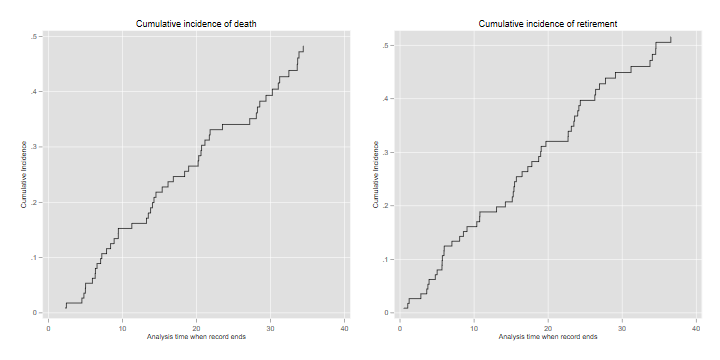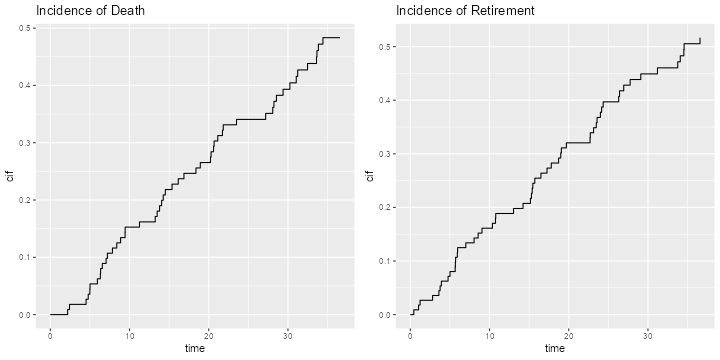### The Status Plot

We now build a nice plot that combines the Kaplan-Meier estimate of overall attrition with the cumulative incidence functions showing atrition by cause. This takes a bit of work, but I think the result is worth the effort.

We use the fact that the survival and incidence functions add up to one, S(t) + I1(t) + I2(t) = 1, so the areas in the plot have boundaries 1, 1 - I1(t), S(t) and 0. The survival can be obtained from Kaplan-Meier or as the complement of the sum of the incidence functions.

In Stata we start by extracting the CIF for death, and fill in the times for other types of events, which are coded as missing. We also add a dummy observation so the plot actually starts at time 0.

```. sort _t

. gen cid = ci if event==1
(63 missing values generated)

. replace cid = cid[_n-1] if missing(cid)

. replace cid = 0 if missing(cid)

. expand 2 in -1 // new observation is at the end
(1 observation created)

. replace _d = . in -1
(1 real change made, 1 to missing)

. replace _t = 0 in -1

. replace km = 1 in -1

. replace cid = 0 in -1

. // We can now compute the boundaries and plot them
. gen b1 = 1

. gen b2 = 1 - cid

. gen b3 = km

. gen b4 = 0

. sort _t

. twoway rarea b1 b2 _t, color("255 160 160") c(J) ///
> ||  rarea b2 b3 _t, color("160 160 255") c(J) ///
> ||  rarea b3 b4 _t, color("160 255 160") c(J) ///
> , legend(off)  xtitle(Years) text(.2 15 "Active") ///
>   text(.5 25 "Retired") text(.8 30 "Died") ///
>   title("Status of Justices by Years since Appointment") ///
>   plotregion(color(white)) graphregion(color(white))

. graph export justices-status.png, width(600) replace
file justices-status.png saved as PNG format

. drop in 1 //remove dummmy
(1 observation deleted)
```

In R we have to deal with the fact that `survfit()` and `cuminc()` arrange their results differently, and that the times do not line up. My solution merges the estimates and uses a simple function to fill the gaps. For the plot itself I found it easier to draw the areas using `polygon()`.

```> # align times and fill gaps
> death <- filter(cifd, cause=="death");
> retirement <- filter(cifd, cause=="retirement")
> dr <- full_join(death, retirement, by = "time") |>
+     select(time, death=3, retirement=5) |> arrange(time)
> fg <- function(x) {
+     for(i in 2:length(x)){
+         if(is.na(x[i])) x[i] <- x[i-1]
+     }
+     x
+ }
> dr <- mutate(dr, death = fg(death), retirement = fg(retirement))
>
> # draw the graph using overlapping polygons
> png("justices-statusr.png", width=600, height=500)
> par(mar=c(2,2,1,1), mgp=c(2 ,.7, 0), tck=-.01, cex=0.75)
> tmax = max(dr\$time)
> plot(c(0, tmax), c(0,1), type="n", xlab="time", ylab="survival")
> polygon(c(0,tmax,tmax,0), c(0,0,1,1),
+     col="#FFA0A0", border="#FFA0A0")
> text(30, .8, "Died")
> polygon(c(dr\$time,tmax,0),c(1 - dr\$death, 0, 0),
+     col = "#A0A0FF", border = "#A0A0FF")
> text(25, .5, "Retired")
> polygon(c(dr\$time,tmax,0),c(1 - dr\$death - dr\$retirement, 0, 0),
+     col="#A0FFA0", border ="#A0FFA0")
> text(15, .2, "Active")
> dev.off()
pdf
2
```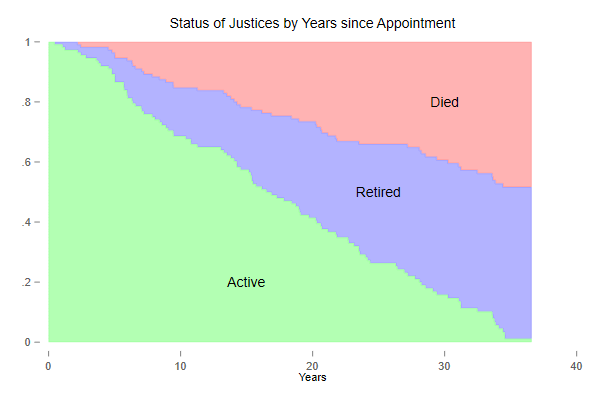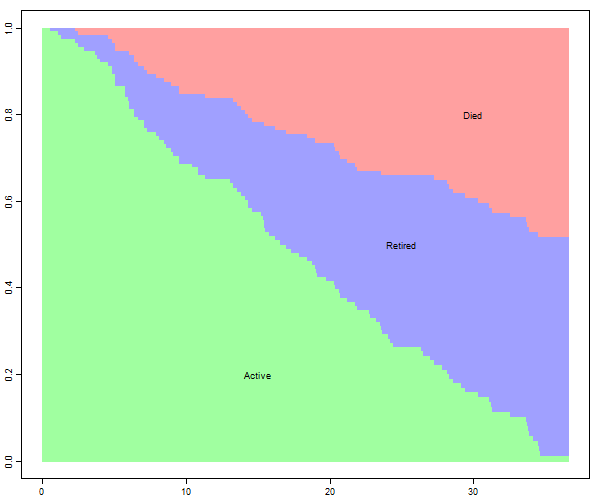The green area is the survival function, showing the probability of remaining in the court, and the other two areas partition attrition into death and retirement, based on the incidence functions. In the long run death and retirement are approximately equally likely.